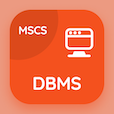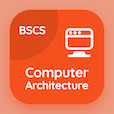Computer Science Courses Online

Digital Logic Design Certification Exam Tests

Digital Logic Design Practice Test 19

# Introduction to Synchronous Sequential Logic Quiz Answers PDF - 19

The Book Introduction to Synchronous Sequential Logic Quiz Questions and Answers, introduction to synchronous sequential logic Quiz MCQs PDF, chapter 14-19 to download online digital logic design course. Study Synchronous Sequential Logics MCQ Questions PDF, introduction to synchronous sequential logic Multiple Choice Questions (MCQ Quiz) for online college degrees. The eBook Introduction to Synchronous Sequential Logic Quiz App Download: introduction to synchronous sequential logic, special characteristics of integrated circuit, basic theorems and properties of boolean algebra, introduction to integrated circuit, adder and subtractors test prep for online college classes.

The Quiz: How many types of sequential circuits have? PDF, "Introduction to Synchronous Sequential Logic" App (Android & iOS) Free with 5, 2, 6, and 7 choices for bachelor's degree in computer science. Practice synchronous sequential logics questions and answers, Google eBook to download free sample for online computer science and engineering.

## Logic Design Quiz Online: Introduction to Synchronous Sequential Logic MCQs - 19

MCQ: How many types of sequential circuits have?

A) 2
B) 5
C) 6
D) 7

MCQ: The maximum number of inputs connected to the gate is called

A) fan
B) fan in
C) fan out
D) out come

MCQ: In Boolean algebra Multiplicative inverse is

A) 0
B) 1
C) 1/a
D) a

MCQ: CMOS stands for

A) Complementary Material Oxide Semiconductor
B) Complementary Metal Oxide Semiconductor
C) Complex Metal Oxide Semiconductor
D) Complex Material Oxide Semiconductor

MCQ: Which of the following expression is true if the output is equal to zero?

A) A>B
B) A
C) A=B
D) A==B

### Mock Tests: Digital Logic Design Course Prep

Download Digital Logic Design Quiz App, DataBase Management System (MCS) MCQ App, and Computer Architecture MCQs App to install for Android & iOS devices. These Apps include complete analytics of real time attempts with interactive assessments. Download Play Store & App Store Apps & Enjoy 100% functionality with subscriptions!